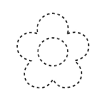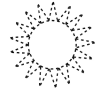# Math in Focus Grade 2 Chapter 18 Practice 1 Answer Key Parts of Lines and Curves

This handy Math in Focus Grade 2 Workbook Answer Key Chapter 18 Practice 1 Parts of Lines and Curves detailed solutions for the textbook questions.

## Math in Focus Grade 2 Chapter 18 Practice 1 Answer Key Parts of Lines and Curves

Trace the correct figures.

Question 1.
Parts of lines only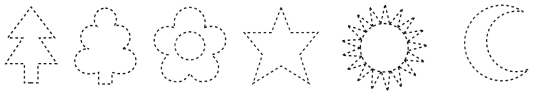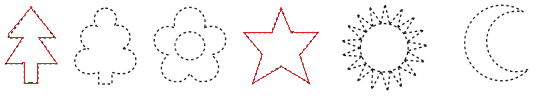Explanation:
I traced the shapes that have parts of lines only.

Question 2.
Curves onlyExplanation:
I traced the shapes that have curves only.

Question 3.
Parts of lines and curves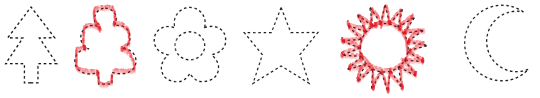Explanation:
I traced the shapes that have parts of lines and curves.

Look at these letters.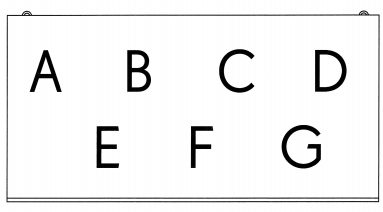Which of these letters have

Question 4.
parts of lines only?
A,E,F

Explanation:
The letters A, E and F have parts of lines only.

Question 5.
curves only?
C

Explanation:
The letter C have only curves.

Question 6.
parts of lines and curves?
B, D, G

Explanation:
The letter B, D and G have parts of lines and curves.

Draw three other letters using parts of lines only.
Question 7.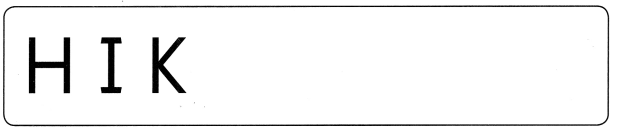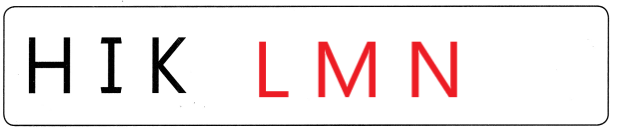Explanation:
I drew 3 letters L, M and N
These letters have parts of lines only.

Draw three other letters using parts of lines and curves.
Question 8.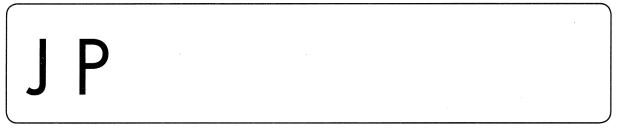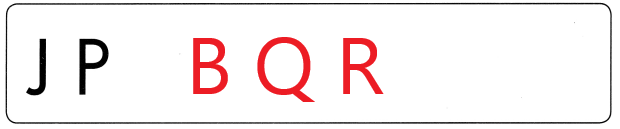Explanation:
I drew 3 letter B, Q and R
These letter have parts of lines and curves.

Julie drew pictures with parts of lines and curves. Count the parts of lines and curves she used.

Example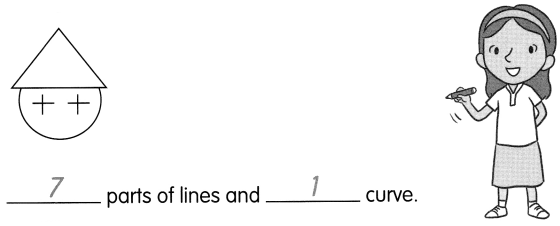Question 9.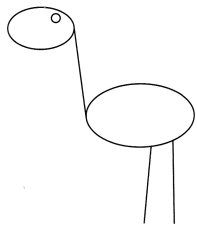___________ parts of lines and __________ curves.
3 parts of lines and 3 curves.

Question 10.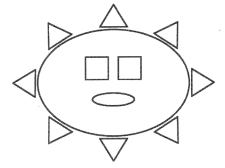___________ parts of lines and __________ curves.
32 parts of lines and 2 curves.

Count the number of parts of lines in each figure. Count the number of curves. Sort the figures into two groups. Sort using the numbers of parts of lines and curves. Color each group a different color.

Question 11.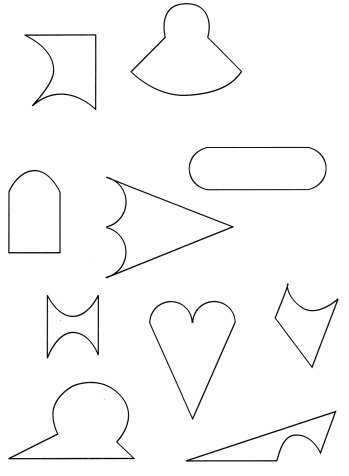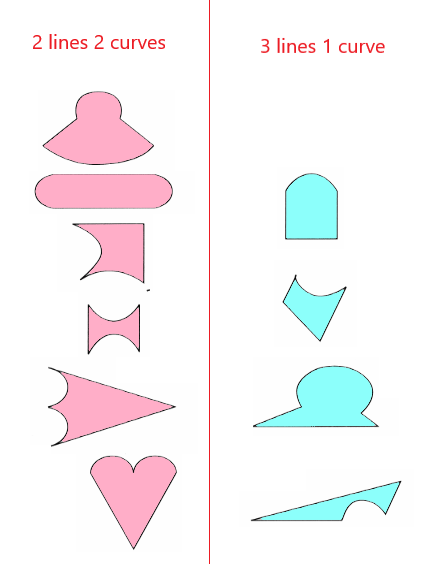Explanation:
I counted the parts of lines and curves
I sorted the shapes into two groups
A group has 2 lines and 2 curves and another group has 3 lines and 1 curve.
I colored the 2 groups with different colors.

Draw a picture with

Question 12.
more than 5 parts of lines.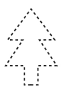Explanation:
There are 11 parts of lines in the above shape
11 is more than 5.

Question 13.
fewer than 8 curves.